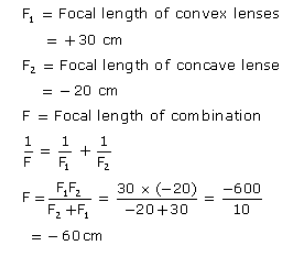# Calculate the focal length of the combination o f a convex lens

Calculate the focal length of the combination o f a convex lens of focal length 30 cm in contact with a concave lens of focal length 20 cm. Is the system a converging or a diverging lens ?The system behaves as a diverging lens.